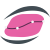#Dew.Stats.Linux 6.0.91

.NET 5.0
`Install-Package Dew.Stats.Linux -Version 6.0.91`
`dotnet add package Dew.Stats.Linux --version 6.0.91`
`<PackageReference Include="Dew.Stats.Linux" Version="6.0.91" />`
For projects that support PackageReference, copy this XML node into the project file to reference the package.
`paket add Dew.Stats.Linux --version 6.0.91`
`#r "nuget: Dew.Stats.Linux, 6.0.91"`
#r directive can be used in F# Interactive, C# scripting and .NET Interactive. Copy this into the interactive tool or source code of the script to reference the package.
```// Install Dew.Stats.Linux as a Cake Addin

// Install Dew.Stats.Linux as a Cake Tool
#tool nuget:?package=Dew.Stats.Linux&version=6.0.91```

Dew Stats Library for .NET Core

Statistical library built on top of Dew Math Linux Library includes among other features:

• Different probability distributions (PDF, CDF and inverse CDF for 36 distributions), random number generators, parameter estimate.
• Descriptive statistics. Histograms, ogives, cumulative sum, nth moments, percentile, range, IQR, mean, median, mode, ranks and more.
• Multivariate Analysis. PCA by using covariance/correlation matrix, PCA residuals, orthogonal rotation of ZScores, Bartlett test for dimensionality and Z-Scores; Classical Multidimensional Scaling, Hotelling T2 test, M-Box test, Item Analysis.
• Design of Experiment. Full Factorial Design, Latin HyperCube design, ...
• Hypothesis testing. Sign test, Wilcoxon Signed Rank test, one-sample t-test, two-sample paired/unpaired t-test, Z-Test, Chi-Squared test, F-Test, Shapiro-Wilks test, Chi-Squared Goodness of Fit test and Shapiro-Francia test; Berra-Jarque, Anderson-Darling, Kolmogorov-Smirnov test, Mann-Whitney U test, LillioeFors Goodness of Fit test, ...
• Regression models. Linear (weighted, unweighted), Multiple linear (weighted, unweighted), Logistic regression, Ridge regression, Poisson regression, General non-linear regression (using the BFGS, Marquardt, Conjugate gradient or Simplex method), one-way and two-way ANOVA, Principal Component Regression.
• Statistical charts. Optional with separate assembly depending on Steema TeeChart.NET: Probabilities plot (Normal, Weibull, QQ), variable control charts ( X, R, S and EWMA), attribute control charts (P, NP, U and C), dot plot, box plot, biplot, error ellipses PLUS all major statistical chart types, supported by Steema's TeeChart (error, barr, error bar, pie, box, scatter, scatter 3D, histogram, Pareto and more).
• Time series analysis. Sample ACF, PACF, exponential smoothing (single, double, triple), support for ARMA/ARIMA models (simulating, forecasting, estimating coefficients by using Yule-Walker, Burg, Innovations and MLE algorithms), ARAR time series model, moving average, memory-shortening filter, Box-Ljung statistics, etc..
• Ready-to-use components. TMtxANOVA (encapsulates ANOVA routines), TMtxMulLinReg (encapsulates multiple linear regression routines), TMtxNonLinReg (encapsulates nonlinear regression routines), TMtxPCA (encapsulates PCA routines), TMtxHypothesisTest (encapsulates hypothesis testing routines), TMtxBinaryTest (encapsulates binary diagonstic test routines), TMtxMDScaling (encapsulates multimensional scaling routines).

Examples

Documentation

Product Versions
.NET net5.0 net5.0-windows net6.0 net6.0-android net6.0-ios net6.0-maccatalyst net6.0-macos net6.0-tvos net6.0-windows
Compatible target framework(s)

### NuGet packages (1)

Showing the top 1 NuGet packages that depend on Dew.Stats.Linux:

Dew.Lab.Studio.Linux

High-performance vectorized numerical math library designed to speed up customer code typically by 10x by using also AVX512 for Linux. Covering multiple areas, Linear Algebra (BLAS and LAPACK), Optimization Methods (Simplex, Marquardt,..), Vectorized math formula evaluator (Matlab/Scilab script), Sparse Matrices (Pardiso, Umfpack, eig-solvers..), Probability distributions, Random number generators, Special (Bessel) functions.

### GitHub repositories

This package is not used by any popular GitHub repositories.